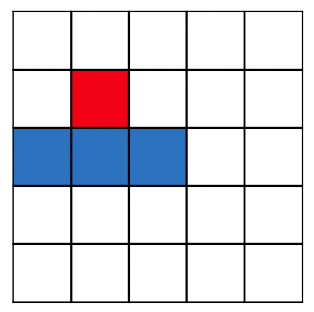# Minimum Cost Path with down, left and right moves allowed

Given a grid with positive integers, I am trying to find a path between the top cell and bottom cell by which total cost incurred is minimum.

How should the code be modified if I want to find the minimum path between any two given points?

And also if I would like to add constraints such that the path can only be formed by moving down one row directly and one left and one right as shown in the picture.
i.e., from a given cell (i, j), cells (i+1, j), (i+1, j-1) and (i+1, j+1)``````# Returns cost of minimum cost path from (0,0) to (m, n) in mat[R][C]
def minCost(cost, m, n):
if (n < 0 or m < 0):
return sys.maxsize
elif (m == 0 and n == 0):
return cost[m][n]
else:
return cost[m][n] + min( minCost(cost, m-1, n-1),
minCost(cost, m-1, n),
minCost(cost, m, n-1) )
``````